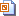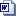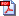Nuffield Mathematics teaching resources are for use in secondary and further education

# Level 1 Spatial techniques

## What is it?

This activity is about recognising and drawing objects from different viewpoints. It is in three sections:

• Identifying objects photographed from plan and elevation views
• Drawing 3D sketches of solids from their plan and elevation views
• Drawing plans and elevations of given objects.

## Convert lengths

Practice in converting between different metric units of length, using exercises on a worksheet, a bingo game, and a dominoes game.

## Volume

Students find the volume of a variety of cuboids in real life contexts.

## Geometrical design

Helping students to recognise geometrical shapes and their properties.

## Measure it!

Estimating lengths and measuring objects using both centimetres and millimetres.

## Plans

Students measure lengths from scale drawings then use ratio scales to find the actual lengths.

## Arranging the furniture

Students design a new layout for the classroom, measuring the furniture, making scaled cut-outs, and placing them on a prepared plan.

## How much will it cost?

Students measure dimensions from scale drawings and find the areas of rectangles and triangles. They then calculate the amount of paint needed to paint a house and its cost.

## Perimeter and area

How much lawn feed is needed? Students find the perimeter and area of rectangles and shapes made from rectangles.

## Drawing shapes in Word

Students learn how to draw and format basic shapes in Word.SlidesStudent sheets Word 2007Student sheets Word 2007 pdfTeacher notes WordTeacher notes pdf